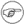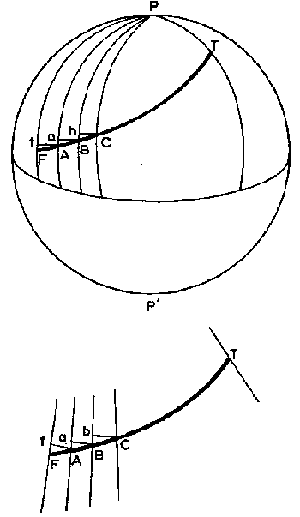## 19.1. Range/Bearing calculations within Debrief

Since Spring 2013, ranges and bearings within Debrief are calculated using the GeoTools GeodeticCalculator derivative of the T.Vincenty approximation:

```        /*
* Solution of the geodetic inverse problem after T.Vincenty.
* Modified Rainsford's method with Helmert's elliptical terms.
* Effective in any azimuth and at any distance short of antipodal.
*
* Latitudes and longitudes in radians positive North and East.
* Forward azimuths at both points returned in radians from North.
*
* Programmed for CDC-6600 by LCDR L.Pfeifer NGS ROCKVILLE MD 18FEB75
* Modified for IBM SYSTEM 360 by John G.Gergen NGS ROCKVILLE MD 7507
* Ported from Fortran to Java by Daniele Franzoni.
*
* Source: ftp://ftp.ngs.noaa.gov/pub/pcsoft/for_inv.3d/source/inverse.for
*         subroutine GPNHRI
*         version    200208.09
*         written by robert (sid) safford
*/
```

LEGACY ALGORITHM In the past, Debrief used the Rhumb-line calculation for the display of measured range-bearing and for the projection of data onto the monitor. Briefly, to convert from real-world to screen coordinates the following procedure was followed:

1. Determine the area represented by the current viewed data in world coordinates

2. Determine the centre of this data area - this is used as the origin of the data

3. For each data point to be plotted, find its range and bearing from the origin

4. Convert this range and bearing into a delta-x and delta-y in screen coordinates

5. Produce a data point in screen coordinates by adding these deltas to the centre of the screen

NoteThe result of this projection algorithm (as with most others) is that whilst the information plotted at the centre horizontal section (mid-latitude) of the screen is an accurate representation, travelling further north and south from it degrades the accuracy. This is negligible in data areas near the equator or where only a small area is covered (less than a couple of hundred nautical miles). With greater data areas, and nearer the poles, the effects are more noticeable, however.

### 19.1.1. LEGACY - The Rhumb-Line formulae

A line on the earth's surface which cuts all meridians at the same angle is called a rhumb line.

With his knowledge of the distance along a parallel of latitude and the departure between two places, the navigator can find the course he must steer in order to follow the rhumb line joining the two places, and also the distance he will travel along whilst doing so. In Figure 19.1, “Rhumb line parts” FT is the rhumb-line divided into a large number of equal parts FA, AB, BC...

Figure 19.1. Rhumb line partsAf, Ba, Cb... are the arcs of parallels drawn through A, B, C ... and the angles at f, a, b ..., are therefore at right-angles. If the divisions of FT are made sufficiently small, the triangles FaF, ABa, BbB ... are themselves small enough to be treated as plane triangles. Also, since the course angle at F, A, B, C, ... remains constant by the definition of a rhumb-line, these small triangles are equal.

### 19.1.2. LEGACY - Short-distance sailing

By the term short-distance sailing is meant the following of a rhumb-line track for a distance not greater than 600'. Within this limiting distance, the navigator can obtain all he wants to know about the track from the three formulae:

```
departure = d.long cos (mean latitude)   (1)
departure = distance sin (course)     (2)
d.lat = distance cos (course)         (3)

The course is given by (2) divided by (3). Thus:
departure / d.lat = tan (course)
```